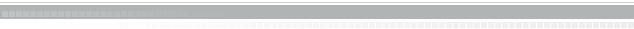KTH MathematicsAdditional material Lecture notes Slides from lecture 1 and Lecture 1. Slides from lecture 2 and Lecture 2. Lecture 3. Lecture 4. Lecture 5. Lecture 6. Lecture 7. Lecture 8 Lecture 9 and 10. Lecture 11 and 12. Lecture 13 and 14. Lecture 15. To Mathematical Statistics To Mathematical Statistics CoursesPublished by: Filip Lindskog Updated: 15/01-2008